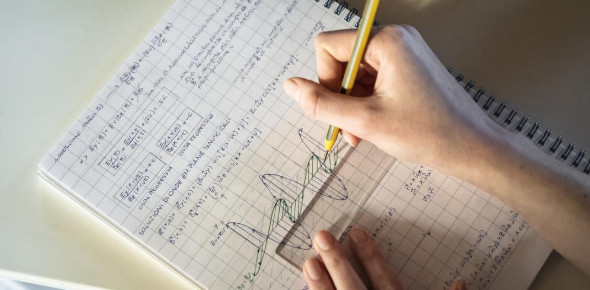15 Questions | Attempts: 307263SettingsAre you in the sixth grade, parent of a sixth-grader, or someone looking for a basic Maths test for practice? If yes, then this Maths quiz for Grade 6 with answers is perfect for you. Answer all the questions correctly and get the perfect score. The quiz below is created to help the quiz taker with the most common sixth-grade mathematics problems. Give it a shot, and remember that practice makes a man perfect. All the best!

• 1.
158=___ + 106. What number will come in the blank to make the number sentence correct?
• A.

52

• B.

152

• C.

158

• D.

264

• 2.
The simplest form of 16/24 is:
• A.

3/5

• B.

2/3

• C.

2/5

• D.

1/3

• 3.
Gaurav has 918 marbles. He wants to make packets of marbles, with nine marbles in each pack. How many packs will he be able to make?
• A.

12

• B.

102

• C.

120

• D.

1062

• 4.
Which of these lies between 6.3 and 6.6?
• A.

6.2

• B.

6.9

• C.

6.05

• D.

6.41

• 5.
A teacher brought some toffees to her class. After giving three toffees each to 15 students who had completed their assignments, she has 60 toffees left with her. How many toffees did she bring to the class?
• A.

15

• B.

45

• C.

78

• D.

105

• 6.
Which of these numbers have only two factors, one and the number itself?
• A.

37+1

• B.

37+37

• C.

37X1

• D.

37X37

• 7.
Meena divides a number by 2. She then divides the answer by 2. This is the same as dividing the original number by:
• A.

1

• B.

2

• C.

4

• D.

It cannot be said as the original number is not known.

• 8.
John has stamps from different countries. 1/3rd of them are India's stamps. If he has 36 Indian stamps, how many stamps does he have in total?
• A.

12

• B.

36

• C.

48

• D.

108

• 9.
1024+1025+___=1025+1025+1025 What number will come in the blank to make the number sentence correct?
• A.

1024

• B.

1025

• C.

1026

• D.

5124

• 10.
Meena has bought six pens. The cost of each pen was between Rs.25 and Rs. 30. Which of these could be the total cost of the pens?
• A.

Rs.55

• B.

Rs.126

• C.

Rs.173

• D.

Rs.330

• 11.
Two angles of a triangle measure 35 degrees and 65 degrees. What is the measure of the third angle of the triangle?
• A.

100 degrees

• B.

80 degrees

• C.

125 degrees

• D.

135 degrees

• 12.
The sum of any three angles of a rectangle is:
• A.

90 degrees

• B.

180 degrees

• C.

270 degrees

• D.

360 degrees

• 13.
The ratio of 500ml to 2 liters is:
• A.

1:4

• B.

1:6

• C.

1:8

• D.

1:10

• 14.
Which of the following is true?
• A.

20 + 0 = 20

• B.

20 - 0 = 0

• C.

20 - 20 - 0 = 20

• D.

20 * 0 = 200

• 15.
The place value of 3 in 1.234:
• A.

3

• B.

3/10

• C.

3/100

• D.

3/1000

## Related TopicsBack to top